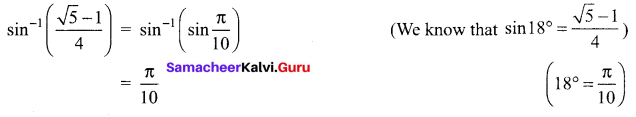# Samacheer Kalvi 12th Maths Solutions Chapter 4 Inverse Trigonometric Functions Ex 4.1

## Tamilnadu Samacheer Kalvi 12th Maths Solutions Chapter 4 Inverse Trigonometric Functions Ex 4.1

Question 1.
Find all the values of x such that
(i) -10π ≤ x ≤ 10π and sin x = 0
(ii) -8π ≤ x ≤ 8π and sin x = -1
Solution:
(i) sin x = 0
⇒ x = nπ
where n = 0, ±1, ±2, ±3, ……., ±10
(ii) sin x = -1
⇒ x = (4n – 1) $$\frac{\pi}{2}$$, n = 0, ±1, ±2, ±3, 4

Question 2.
Find the period and amplitude of
(i) y = sin 7x
(ii) y = -sin($$\frac{1}{3}$$x)
(iii) y = 4 sin(-2x)
Solution:
(i) y = sin 7x
Period of the function sin x is 2π
Period of the function sin 7x is $$\frac{2 \pi}{7}$$
The amplitude of sin 7x is 1.

(ii) y = -sin$$\frac{1}{3}$$x
Period of sin x is 2π
So, period of sin$$\frac{1}{3}$$x is 6π and the amplitude is 1.

(iii) y = 4 sin(-2x) = -4 sin 2x
Period of sin x is 2π
π Period of sin 2x is π and the amplitude is 4.Question 3.
Sketch the graph of y = sin($$\frac{1}{3}$$x) for 0 ≤ x < 6π.
Solution:
The period of sin($$\frac{1}{3}$$x) is 6π and the amplitude is 1.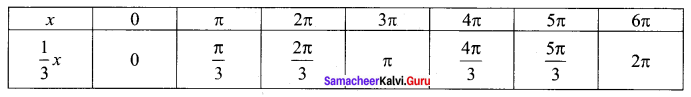The graph is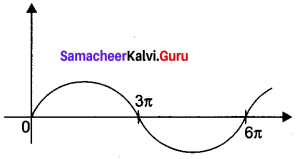Question 4.
Find the value of
(i) $$\sin ^{-1}\left(\sin \left(\frac{2 \pi}{3}\right)\right)$$
(ii) $$\sin ^{-1}\left(\sin \left(\frac{5 \pi}{4}\right)\right)$$
Solution: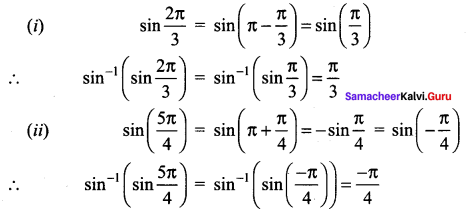Question 5.
For that value of x does sin x = sin-1 x?
Solution:
sin x = sin-1 x is possible only when x = 0 (∵ x ∈ R)

Question 6.
Find the domain of the following
(i) $$f(x)=\sin ^{-1}\left(\frac{x^{2}+1}{2 x}\right)$$
(ii) $$g(x)=2 \sin ^{-1}(2 x-1)-\frac{\pi}{4}$$
Solution:
(i) $$f(x)=\sin ^{-1}\left(\frac{x^{2}+1}{2 x}\right)$$
The range of sin-1 x is -1 to 1
$$-1 \leq \frac{x^{2}+1}{2 x} \leq 1$$
⇒ $$\frac{x^{2}+1}{2 x} \geq-1$$ or $$\frac{x^{2}+1}{2 x} \leq 1$$
⇒ x2 + 1 ≥ -2x or x2 + 1 ≤ 2x
⇒ x2 + 1 + 2x ≥ 0 or x2 + 1 – 2x ≤ 0
⇒ (x+ 1)2 ≥ 0 or (x – 1)2 ≤ 0 which is not possible
⇒ -1 ≤ x ≤ 1 or
(ii) $$g(x)=2 \sin ^{-1}(2 x-1)-\frac{\pi}{4}$$
-1 ≤ (2x – 1) ≤ 1
0 ≤ 2x ≤ 2
0 ≤ x ≤ 1
x ∈ [0, 1]Question 7.
Find the value of $$\sin ^{-1}\left(\sin \frac{5 \pi}{9} \cos \frac{\pi}{9}+\cos \frac{5 \pi}{9} \sin \frac{\pi}{9}\right)$$
Solution: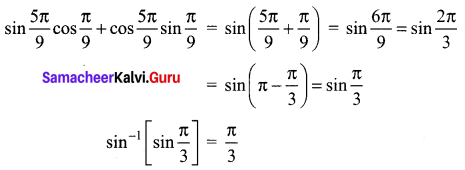### Samacheer Kalvi 12th Maths Solutions Chapter 4 Inverse Trigonometric Functions Ex 4.1 Additional Problems

Question 1.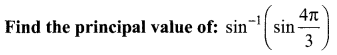Solution: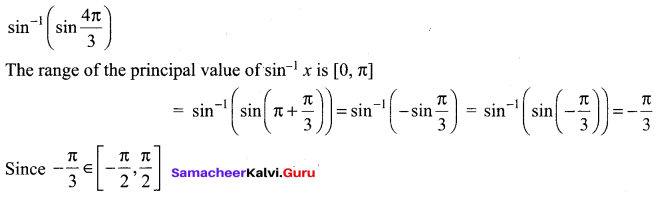Question 2.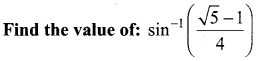Solution: